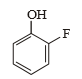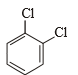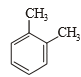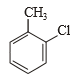Among the following isostructural compounds, which one has highest lattice energy ?

(1) LiCl

(2) MgO

(3) NaCl

(4) LiF

Concept Questions :-

Lattice/Hydration Energy
High Yielding Test Series + Question Bank - NEET 2020

Difficulty Level:

sbonded molecule MX3 is T-shaped. The number of non-bonding pairs of electrons is :

(1) 0

(2) 2

(3) 1

(4) Can be predicted only if atomic number of M is known,

Concept Questions :-

Hybridisation
High Yielding Test Series + Question Bank - NEET 2020

Difficulty Level:

In a chemical change from  the hybrid state of P changes from:

(1) sp2 to sp3

(2) sp3 to sp2

(3) sp3 to sp3d

(4) sp3 to dsp

Concept Questions :-

Hybridisation
High Yielding Test Series + Question Bank - NEET 2020

Difficulty Level:

The geometrical arrangement and shape of ${\mathrm{I}}_{3}^{-}$ are respectively

1. Trigonal bipyramidal geometry, linear shape

2. Hexagonal structure, linear shape

3. Triangular planar geometry, triangular shape

4. Tetrahedral geometry, pyramidal shape

Concept Questions :-

Hybridisation
High Yielding Test Series + Question Bank - NEET 2020

Difficulty Level:

which is the incorrect about bond angles ?

1. NH3 > NF3

2. NF3 <  PF3

3. NH3 > PH3

4. NH3 > H2O

Concept Questions :-

Polarity
High Yielding Test Series + Question Bank - NEET 2020

Difficulty Level:

Which molecules/ions are most paramagnetic?

1. B2

2. C2

3. ${\mathrm{O}}_{2}^{+}$

4. ${\mathrm{O}}_{2}^{-}$

Concept Questions :-

M.O.T
High Yielding Test Series + Question Bank - NEET 2020

Difficulty Level:

In which case observed dipole moment is greater than theoretical dipole moment?

1.2.3.4.Concept Questions :-

Polarity
High Yielding Test Series + Question Bank - NEET 2020

Difficulty Level:

Which has linear shape but not sp hibridisation ?

(1) ${\mathrm{XeF}}_{2}$

(2) ${\mathrm{I}}_{3}^{-}$

(3) ${\mathrm{CO}}_{2}$

(4) Both 1 and 2

Concept Questions :-

Hybridisation
High Yielding Test Series + Question Bank - NEET 2020

Difficulty Level:

Which of the following structure is most expected for the molecule XeOF4 ?

(1) Tetrahedral

(2) Square pyramid

(3) Square planar

(4) Octahedral

Concept Questions :-

Hybridisation
High Yielding Test Series + Question Bank - NEET 2020

Difficulty Level:

Which has highest bond energy ?

1. CO

2. CO+

3. N2

4. ${\mathrm{N}}_{2}^{+}$

Concept Questions :-

M.O.T